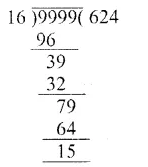# RS Aggarwal Solutions Class 6 Chapter 3 Whole Numbers Exercise 3E

In this chapter, we provide RS Aggarwal Solutions for Class 6 Chapter 3 Whole Numbers Exercise 3E for English medium students, Which will very helpful for every student in their exams. Students can download the latest RS Aggarwal Solutions for Class 6 Chapter 3 Whole Numbers Exercise 3E pdf, free RS Aggarwal Solutions Class 6 Chapter 3 Whole Numbers Exercise 3E book pdf download. Now you will get step by step solution to each question.

### RS Aggarwal Class 6 Chapter 3 Whole Numbers Ex 3E Download PDF

Question 1.
Solution:
(i) By actual division, we haveQuestion 2.
Solution:
(i) By actual division, we haveQuestion 3.
Solution:
(i) We know that any number (non-zero) divided by 1 gives the number itself
65007 ÷ 1 = 65007
(ii) We know that 0 divided by any natural number gives 0
0 ÷ 879 = 0
(iii) 981 + 5720 ÷ 10 = 981 + (5720 ÷ 10)
= 981 + 572
= 1553
(iv) 1507 – 625 ÷ 25 = 1507 – (625 ÷ 25)
= 1507 – 25
= 1482
(v) 32277 ÷ (648 – 39)
= 32277 ÷ 60932277 ÷ (648 – 39) = 53
(vi) 1573 ÷ 1573 – 1573 ÷ 1573
= (1573 ÷ 1573) – (1573 ÷ 1573)
= 1 – 1
= 0

Question 4.
Solution:
We have n ÷ n = n
let n = 1, 1 ÷ 1 = 1
1 = 1
which is true
∴ Hence 1 is the required whole number.

Question 5.
Solution:
Product of two numbers = 504347
One number = 317
Other number = 504347 ÷ 317∴ Other number = 1591

Question 6.
Solution:
Here Dividend = 59761, Quotient = 189
∴ Remainder = 37
We know that Dividend = Divisor x Quotient + Remainder
59761 = Divisor x 189 + 37
59761 – 37 = Divisor x 189
59724 = Divisor x 189
Divisor x 189 = 59724
∴ Divisor = 59724 ÷ 189∴ Divisor = 59724 ÷ 189 = 316

Question 7.
Solution:
Here dividend = 55390,
Divisor = 299 and Remainder = 75
By division algorithm, we have
Dividend = Quotient x Divisor + Remainder
55390 = Quotient x 299 + 75
55390 – 75 = Quotient x 299
55315 = Quotient x 299
Quotient x 299 = 55315
Quotient = 55315 ÷ 299∴ Required quotient = 185

Question 8.
Solution:
On dividing 13601 by 87, we haveIt is clear that if we subtract 29 from 13601, the resulting number will be exactly divisible by 87.
∴ The required least number = 29.

Question 9.
Solution:
Here dividend = 1056, Divisor = 23
By actual division, we haveIt is clear that if we add 2 to 21, it will become 23 which is divisible by 23.
∴ Required least number = 2.

Question 10.
Solution:
Greatest 4-digit number = 9999On, dividing by 16, we get remainder as 15
∴ The required largest 4-digit number = 9999 – 15
= 9984

Question 11.
Solution:
Largest number of 5-digits = 99999
On dividing 99999 by 653, we have∴ Quotient = 153, Remainder = 90
Check : By division algorithm Dividend = Divisor x Quotient + Remainder
= 653 x 153 + 90
= 99909 + 90
= 99999

Question 12.
Solution:
The least 6-digit number = 100000
On dividing 100000 by 83, we haveIt is clear that if we add 15 to 68, it will become 83 which is divisible by 83.
∴ Required least 6-digit number = 100000 + 15
= 100015

Question 13.
Solution:
Cost of 1 dozen bananas = Rs. 29
Bananas can be purchase in Rs. 13921392 ÷ 29
= 48 dozens

Question 14.
Solution:
Total number of trees = 19625
Total number of rows = 157
Number of trees in each row = 19625 ÷ 157∴ Number of trees in each row = 125

Question 15.
Solution:
Total population of the town = 517530
Since there is one educated person out of 15
Total number of educated persons in the town = 517530 ÷ 15∴ Total number of educated persons in the town = 34502.

Question 16.
Solution:
Cost of 23 colour TV sets = Rs. 570055
Cost of 1 colour TV set∴ Cost of 1 color TV set
= Rs. 570055 ÷ 23
= Rs. 24785

All Chapter RS Aggarwal Solutions For Class 6 Maths

—————————————————————————–

All Subject NCERT Exemplar Problems Solutions For Class 6

All Subject NCERT Solutions For Class 6

*************************************************

I think you got complete solutions for this chapter. If You have any queries regarding this chapter, please comment on the below section our subject teacher will answer you. We tried our best to give complete solutions so you got good marks in your exam.

If these solutions have helped you, you can also share rsaggarwalsolutions.in to your friends.